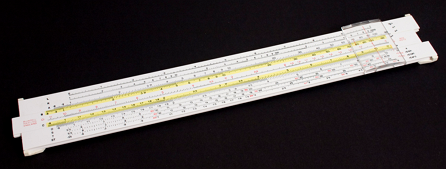# Log Number

Algebra Level 2Suppose a real number $A$ satisfy the inequality $8^{21} < A < 8^{22}$ and $\log _{ 2 }{ A }$ is an even number, find the value of $\log _{ 4 }{ A }$.

×